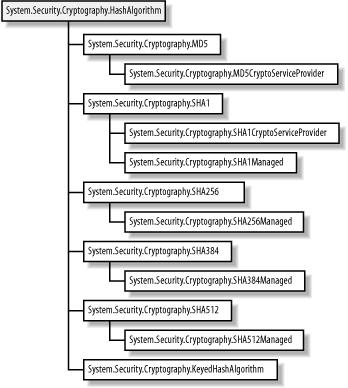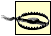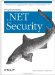# 13.2 Programming Hashing Algorithms

The .NET Framework represents all hashing algorithms with the abstract System.Security.Cryptography.HashAlgorithm class. An abstract class represents each specific algorithm, extended by individual implementation classes, as shown in Figure 13-3. This approach supports selecting between several implementations of the same algorithm.

##### Figure 13-3. The .NET Framework class hierarchy for hashing algorithmsThere are two types of implementation class included with the .NET Framework. Those whose names end with Managed (for example, SHA1Managed) were written in a managed .NET language (for example, C# or Visual Basic .NET). Those classes whose names end in CryptoServiceProvider (for example, SHA1CryptoServiceProvider) rely on the Windows Crypto API. Where there is a choice between a managed implementation and a Crypto API implementation, our (unscientific) testing shows that the Crypto API versions are slightly faster and consume less system memory.

## The Windows Crypto API

The Crypto API is a component of the Windows operating system that contains cryptographic functionality. Many of the .NET cryptographic classes rely on features of the Crypto API for tasks such as key management or providing implementations of algorithms. As a .NET programmer, you do not need to have an understanding of the details of the Crypto API. We do not explore the Crypto API in this book; for more information, consult the Windows API documentation.

#### 13.2.1 The HashAlgorithm Class

The design of the HashAlgorithm class makes it very simple to generate hash codes for any of the hashing algorithms that the .NET Framework supports. In this section, we show you how to create an instance of a given hashing algorithm and the techniques used to create hash codes for different types of data. Table 13-2 lists the members of the HashAlgorithm class.

##### Table 13-2. Members of the HashAlgorithm Class

Member

Description

Properties

Hash

This property returns the value of the computed hash code. It is not commonly used. See the following sections for instructions on using the ComputeHash method to create hash codes.

HashSize

This property returns the size of the hash code (in bits) that the hashing algorithm produces. See Table 13-1 for details of the hashing algorithms implemented in the .NET Framework and the sizes of the hash codes that they produce.

CanReuseTransform

These properties are from the ICryptoTranform interface, which is not directly related to hashing, but is implemented by the HashAlgorithm class. See Chapter 14 for more information.

CanTransformMultipleBlocks

InputBlockSize

OutputBlockSize

Methods

Create

This static method creates a new instance of the HashAlgorithm class by name. See Section 13.2.2 for further details.

ComputeHash

The ComputeHash method is used to create hash codes from byte arrays and data streams. See Section 13.2.3 and Section 13.2.4 in this chapter for full details.

Initialize

This is used by algorithm-implementation classes to initialize their state before use.

Clear

This releases the resources held by the HashAlgorithm instance.

TransformBlock

These methods are from the ICryptoTransform interface, which is not directly related to hashing, but is implemented by the HashAlgorithm class. See Chapter 14 for more information.

TransformFinalBlock

#### 13.2.2 Instantiating the Algorithm

The first step toward creating a hash code is to create an instance of an implementation class for the algorithm that you want to use. The simplest way of doing this is to use the name of the class directly:

`# C# SHA1Managed x_hash_alg = new SHA1Managed(  ); # Visual Basic .NET Dim x_hash_alg As SHA1Managed = New SHA1Managed(  )`

This statement creates an instance of the managed-code implementation of the SHA-1 algorithm. This is the clearest and most direct approach to instantiating hashing algorithms. Another approach is to use the static HashAlgorithm.Create method to instantiate the class indirectly:

`# C# HashAlgorithm x_hash_alg = HashAlgorithm.Create("SHA1"); # Visual Basic .NET Dim x_hash_alg As HashAlgorithm = HashAlgorithm.Create("SHA1")`

The Create method instantiates an algorithm based on the value of the string argument. Table 13-3 lists the mapping between string values and implementation classes.

##### Table 13-3. Mapping string values to algorithm classes

String value

Algorithm-implementation class

MD5

MD5CryptoServiceProvider

System.Security.Cryptography.MD5

MD5CryptoServiceProvider

SHA

SHA1CryptoServiceProvider

SHA1

SHA1CryptoServiceProvider

System.Security.Cryptography.SHA1

SHA1CryptoServiceProvider

SHA256

SHA256Managed

SHA-256

SHA256Managed

System.Security.Cryptography.SHA256

SHA256Managed

SHA384

SHA384Managed

SHA-384

SHA384Managed

System.Security.Cryptography.SHA384

SHA384Managed

SHA512

SHA512Managed

SHA-512

SHA512Managed

System.Security.Cryptography.SHA512

SHA512ManagedIf you do not supply a string as an argument to the Create method or the string is not one of the values listed in Table 13-3, then the System.Security.Cryptography.SHA1CryptoServiceProvider algorithm will be used as a default.

This approach is less direct but does have a number of benefits. First, we only have to change the value of the string to change the algorithm that is used. Second, the systems administrator can alter the mappings listed in Table 13-3 by editing the .NET configuration files, potentially allowing a customer to use a different algorithm for specific tasks. For example, a customer may want to use specialized hardware to generate hash codes rather than relying on the default software implementations. We recommend that you use the HashAlgorithm.Create method for the flexibility that it offers.

#### 13.2.3 Hashing Data from Memory

The simplest way to generate a hash code is to use data held in memory. The overloaded ComputeHash method is able to create a hash code from a byte array, as shown by the following signature:

`# C# public byte[] ComputeHash(    byte[] buffer );  # Visual Basic .NET Overloads Public Function ComputeHash( _    ByVal buffer(  ) As Byte _ ) As Byte(  )`

The ComputeHash returns a byte array that contains the hash code for the message data. The following class demonstrates how to create a hash code for a String value; note that you should use the System.Text.Encoding class to convert the string to a byte array:

`# C# using System; using System.Text; using System.Security.Cryptography; class StringHash {         static void Main(string[] args) {                  // define the string that we will         // create a hash code for         String x_str = "Programming .NET Security";         // create a byte array from the string         byte[] x_message_data = Encoding.Default.GetBytes(x_str);         // create an instance of the MD5 hashing algorithm         HashAlgorithm x_hash_alg = HashAlgorithm.Create("MD5");         // obtain the hash code from the HashAlgorithm by          // using the ComputeHash method         byte[] x_hash_code = x_hash_alg.ComputeHash(x_message_data);         // print out the hash code to the console         foreach (byte x_byte in x_hash_code) {             Console.Write("{0:X2} ", x_byte);         }         } } # Visual Basic .NET Imports System Imports System.Text Imports System.Security.Cryptography Module StringHash      Sub Main(  )         ' define the string that we will         ' create a hash code for         Dim x_str As String = "Programming .NET Security"         ' create a byte array from the string         Dim x_message_data(  ) As Byte = Encoding.Default.GetBytes(x_str)         ' create an instance of the MD5 hashing algorithm         Dim x_hash_alg As HashAlgorithm = HashAlgorithm.Create("MD5")         ' obtain the hash code from the HashAlgorithm by          ' using the ComputeHash method         Dim x_hash_code(  ) As Byte = x_hash_alg.ComputeHash(x_message_data)         ' print out the hash code to the console         Dim x_byte As Byte         For Each x_byte In x_hash_code             Console.Write("{0:X2} ", x_byte)         Next     End Sub End Module`

The output from this example is:

`E1 62 9F C2 96 85 C3 A4 5B 94 97 57 D8 9C 65 78`

To make the hash code easier to read, convert each byte to a hexadecimal value and print a space between each one. When you used the ComputeHash method in the previous example, you wanted to process an entire byte array; however, the HashAlgorithm class includes a different version of the method, which can process a region of a byte array:

`# C# public byte[] ComputeHash(    byte[] buffer,    int offset,    int count ); # Visual Basic .NET Overloads Public Function ComputeHash( _    ByVal buffer(  ) As Byte, _    ByVal offset As Integer, _    ByVal count As Integer _ ) As Byte(  )`

#### 13.2.4 Hashing Streamed Data

You can also create a hash code from a stream of data; this is useful for processing datafiles or for reading data from a network connection. The HashAlgorithm class contains an overloaded version of the ComputeHash method for working with streams:

`# C# public byte[] ComputeHash(    Stream inputStream ); # Visual Basic .NET Overloads Public Function ComputeHash( _    ByVal inputStream As Stream _ ) As Byte(  )`

The following class demonstrates how to create a hash code using a stream, in this case streaming data from a file called myfile.txt, which contains the string "Programming .NET Security":

`# C# using System; using System.IO; using System.Security.Cryptography; class StreamHash {     static void Main(string[] args) {                  // create the file stream          Stream x_stream = new FileStream("mydata.txt", FileMode.Open);         // create an instance of the MD5 hashing algorithm         HashAlgorithm x_hash_alg = HashAlgorithm.Create("MD5");         // obtain the hash code from the HashAlgorithm by          // using the ComputeHash method         byte[] x_hash_code = x_hash_alg.ComputeHash(x_stream);         // print out the hash code to the console         foreach (byte x_byte in x_hash_code) {             Console.Write("{0:X2} ", x_byte);         }         // close the stream         x_stream.Close(  );     } } # Visual Basic .NET Imports System Imports System.IO Imports System.Security.Cryptography Module StreamHash     Sub Main(  )         ' create the file stream          Dim x_stream As Stream = New FileStream("mydata.txt", FileMode.Open)         ' create an instance of the MD5 hashing algorithm         Dim x_hash_alg As HashAlgorithm = HashAlgorithm.Create("MD5")         ' obtain the hash code from the HashAlgorithm by          ' using the ComputeHash method         Dim x_hash_code(  ) As Byte = x_hash_alg.ComputeHash(x_stream)         ' print out the hash code to the console         Dim x_byte As Byte         For Each x_byte In x_hash_code             Console.Write("{0:X2} ", x_byte)         Next         ' close the stream         x_stream.Close(  )     End Sub End Module`

As you might expect, the output from the example, shown below, is the same hash code produced by our first example:

`E1 62 9F C2 96 85 C3 A4 5B 94 97 57 D8 9C 65 78`

#### 13.2.5 Validating Hash Codes

Now that you have seen how to create hash codes (playing the role of Alice), we will show you how to validate them, playing the role of Bob. Remember that Bob receives a hash code and a message from Alice. He generates his own hash code and checks that it matches the one that he received. Example 13-1 shows a simple approach to validating hash codes:

##### Example 13-1. Validating a hash code
`# C# using System; using System.Text; using System.Security.Cryptography; class HashCodeValidation {     private static byte[] ParseHashCodeString(string p_hash_code_string) {         // split the hash code on spaces         string[] x_elements = p_hash_code_string.Split(' ');         // create a byte array to hold the elements         byte[] x_hash_code_array = new byte[x_elements.Length];         // parse each string element into a byte         for (int i = 0; i < x_elements.Length; i++) {             x_hash_code_array[i] = Byte.Parse(x_elements[i],                  System.Globalization.NumberStyles.HexNumber);         }         // return the byte array         return x_hash_code_array;     }     private static bool CompareHashCodes(byte[] x_hash_code1,          byte[] x_hash_code2) {         // check that the hash codes are the same length         if (x_hash_code1.Length == x_hash_code2.Length) {             // run through the hash code and check              // each value in turn             for (int i = 0; i < x_hash_code1.Length; i++) {                 if (x_hash_code1[i] != x_hash_code2[i]) {                     // the byte at this location is different                     // in each hash code                     return false;                 }             }             // the hash codes are the same             return true;         } else {             // the hash codes contain different numbers of             // bytes and so cannot be the same             return false;         }     }     public static bool ValidateHashCode(string p_hash_algorithm,          string p_hash_string, byte[] p_data) {         // parse the hash code string into a byte array         byte[] x_hash_code = ParseHashCodeString(p_hash_string);         // create the hashing algorithm object using the          // name argument         HashAlgorithm x_hash_alg = HashAlgorithm.Create(p_hash_algorithm);         // compare the hash codes         return CompareHashCodes(x_hash_code, x_hash_alg.ComputeHash(p_data));     }     static void Main(string[] args) {             // define the message data that Alice sent to Bob         string x_message_data = "Programming .NET Security";         // covert the message data into a byte array         byte[] x_message_bytes = Encoding.Default.GetBytes(x_message_data);         // define the hash code that Alice sent to Bob         string x_hash_string =             "E1 62 9F C2 96 85 C3 A4 5B 94 97 57 D8 9C 65 78";         // define the name of the hashing algorithm that Alice used         string x_algorithm_name = "MD5";         // validate the hash code and write out the result         bool x_result = ValidateHashCode(x_algorithm_name,              x_hash_string, x_message_bytes);         if (x_result) {             Console.WriteLine("The hash code is valid");         } else {             Console.WriteLine("The hash code is invalid");         }     } } # Visual Basic .NET Imports System.Security.Cryptography Imports System.Text Module HashCodeValidation     Public Function ParseHashCodeString(ByVal p_hash_code_string _     As String) As Byte(  )         ' split the hash code on spaces         Dim x_elements(  ) As String = p_hash_code_string.Split(" "c)         ' create a byte array to hold the elements         Dim x_hash_code_array(  ) As Byte = New Byte(x_elements.Length - 1) {}         ' parse each string element into a byte         Dim i As Integer         For i = 0 To x_elements.Length - 1 Step i + 1             x_hash_code_array(i) = Byte.Parse(x_elements(i), _             System.Globalization.NumberStyles.HexNumber)         Next         ' return the bye array         Return x_hash_code_array     End Function     Private Function CompareHashCodes(ByVal x_hash_code1(  ) As Byte, _     ByVal x_hash_code2(  ) As Byte) As Boolean         ' check that the hash codes are the same length         If x_hash_code1.Length = x_hash_code2.Length Then             ' run through the hash code and check              ' each value in turn             Dim i As Integer             For i = 0 To x_hash_code1.Length - 1 Step i + 1                 If x_hash_code1(i) <> x_hash_code2(i) Then                     ' the byte at this location is different                     ' in each hash code                     Return False                 End If             Next             ' the hash codes are the same             Return True         Else             ' the hash codes contain different numbers of             ' bytes and so cannot be the same             Return False         End If     End Function     Public Function ValidateHashCode(ByVal p_hash_algorithm As String, _     ByVal p_hash_string As String, ByVal p_data(  ) As Byte) As Boolean         ' parse the hash code string into a byte array         Dim x_hash_code(  ) As Byte = ParseHashCodeString(p_hash_string)         ' create the hashing algorithm object using the          ' name argument         Dim x_hash_alg As HashAlgorithm = HashAlgorithm.Create(p_hash_algorithm)         ' compare the hash codes         Return CompareHashCodes(x_hash_code, x_hash_alg.ComputeHash(p_data))     End Function     Sub Main(  )         ' define the message data that Alice sent to Bob         Dim x_message_data As String = "Programming .NET Security"         ' covert the message data into a byte array         Dim x_message_bytes(  ) As Byte = Encoding.Default.GetBytes(x_message_data)         ' define the hash code that Alice sent to Bob         Dim x_hash_string As String = _             "E1 62 9F C2 96 85 C3 A4 5B 94 97 57 D8 9C 65 78"         ' define the name of the hashing algorithm that Alice used         Dim x_algorithm_name As String = "MD5"         ' validate the hash code and write out the result        Dim x_result As Boolean = ValidateHashCode(x_algorithm_name, _        x_hash_string, x_message_bytes)         If (x_result) Then             Console.WriteLine("The hash code is valid")         Else             Console.WriteLine("The hash code is invalid")         End If     End Sub End Module`

The first step in validating the hash code is to parse the information that Bob has received. The format we have used to express the hash codes is the most commonly used, but you may encounter other formats.

The ParseHashCodeString method accepts a string containing a formatted hash code and produces a byte array. The CompareHashCodes method takes care of comparing two arrays of bytes to ensure that they contain the same hash code.

The ValidateHashCode method uses the other two methods to validate a hash code, taking three arguments:

1. The name of the algorithm that Alice used to create the hash code

2. The hash code Alice created, expressed as a string of hexadecimal bytes

3. The message from Alice, expressed as a string

Parse the hash code string into a byte array, and then create an instance of the HashAlgorithm class based on the algorithm name. Assume that Alice and Bob agree on the algorithm that was used to create the hash codes, or that Alice picks one and indicates which algorithm she has used by sending some information along with the message. Notice how you cannot create an instance of any supported algorithm using the same code statements; this is another benefit of instantiating algorithms by name.

Finally, calculate a hash code for the message data and compare it to the one that Bob received from Alice. The example includes a Main method to demonstrate how to validate the hash code that you created previously.Programming .Net Security
ISBN: 0596004427
EAN: 2147483647
Year: 2005
Pages: 346

Similar book on Amazon

flylib.com © 2008-2017.
If you may any questions please contact us: flylib@qtcs.net